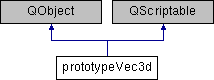Developer Documentation
prototypeVec3d Class Reference

`#include <OpenFlipper/Scripting/scriptPrototypes/prototypeVec3d.hh>`

Inheritance diagram for prototypeVec3d:## Public Slots

void multiply (QScriptValue _scalar)
Multiplies the given vector with the scalar. More...

Adds another vector to this vector. More...

void sub (QScriptValue _vector)
Subtracts another vector from this vector. More...

void zero ()
Resets all components of the vector to zero. More...

QScriptValue sprod (QScriptValue _vector)
Calculate scalar product. More...

QScriptValue norm ()
Calculate the Euclidean norm of the vector. More...

QScriptValue sqrnorm ()
Calculate the squared Euclidean norm of the vector. More...

void normalize ()
Normalize the vector.

QString toString () const

## Public Member Functions

prototypeVec3d (QObject *parent=0)

## Detailed Description

This class wraps the Vec3d to the scripting language

Definition at line 105 of file prototypeVec3d.hh.

## Member Function Documentation

 void prototypeVec3d::add ( QScriptValue _vector )
slot

Adds another vector to this vector.

Parameters
 _vector Vector that should be added

Definition at line 85 of file prototypeVec3d.cc.

 void prototypeVec3d::multiply ( QScriptValue _scalar )
slot

Multiplies the given vector with the scalar.

Parameters
 _scalar Scalar value that gets multiplied

Definition at line 79 of file prototypeVec3d.cc.

 QScriptValue prototypeVec3d::norm ( )
slot

Calculate the Euclidean norm of the vector.

Returns
Norm of the vector

Definition at line 111 of file prototypeVec3d.cc.

 QScriptValue prototypeVec3d::sprod ( QScriptValue _vector )
slot

Calculate scalar product.

Parameters
 _vector Second vector for scalar product (Can be the vector itself)
Returns
Scalar product value

Definition at line 104 of file prototypeVec3d.cc.

 QScriptValue prototypeVec3d::sqrnorm ( )
slot

Calculate the squared Euclidean norm of the vector.

Returns
Squared norm of the vector

Definition at line 117 of file prototypeVec3d.cc.

 void prototypeVec3d::sub ( QScriptValue _vector )
slot

Subtracts another vector from this vector.

Parameters
 _vector Vector that should be subtracted

Definition at line 92 of file prototypeVec3d.cc.

 void prototypeVec3d::zero ( )
slot

Resets all components of the vector to zero.

Definition at line 98 of file prototypeVec3d.cc.

The documentation for this class was generated from the following files: